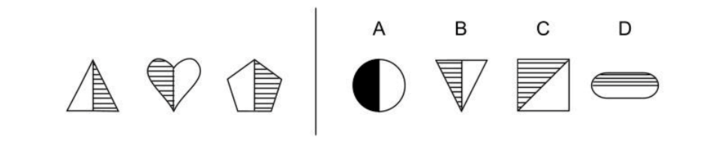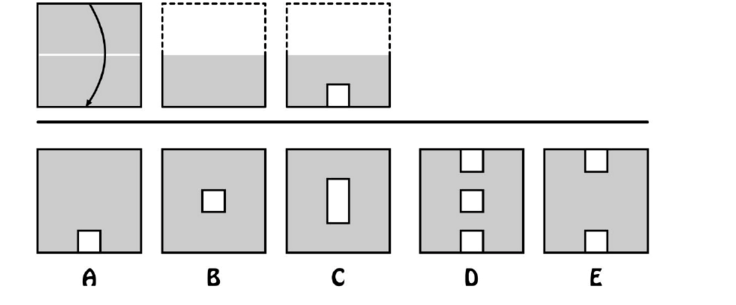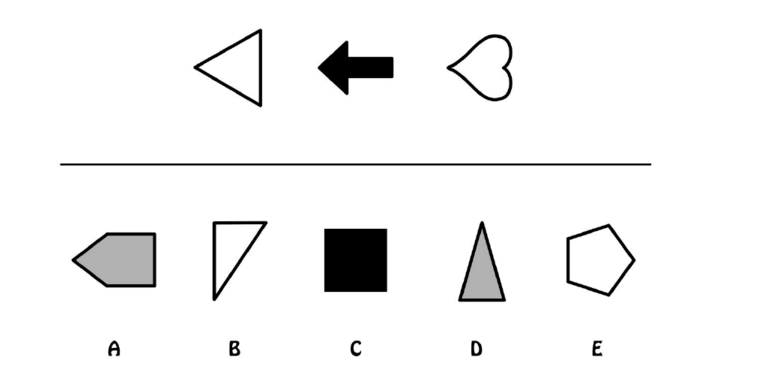Start Now with 100 Free Questions
View MoreHide Information

# CogAT Non-Verbal Battery

The CogAT nonverbal battery is designed to measure a student’s problem solving and reasoning abilities through the use of visual and spatial based questions. This section of the CogAT is unique in that students do not rely on the use of words, but instead geometric shapes and figures. The Non-verbal battery may present as being the most unfamiliar to students, due to the fact that many of the questions are not formally taught in the classroom. This battery consists of figure matrices, paper folding, and figure classification questions.

## Figure Matrices

These figure analogies require the same thought processes as picture and number analogies. Still, instead of verbal or quantitative concepts, students are required to identify relationships between spatial forms. By studying and identifying distinct points of relation between previous figures, students can assess possible answer choices.

Sample Question- The first 3 figures on the left relate to each other in some way. Find a figure on the right of the line that goes with the first 3 figures in exactly the same way.Answer- B- Vertical line divides figure into horizontal stripes and solid white.

## Paper Folding

Students must imagine what will happen to a piece of paper that is folded, then cut or hole- punched in some way, and then unfolded. The testing.com website has a fun, animated game to help students with this subtest. This section consists of 10 questions and takes approximately 10 minutes to complete.

Sample Question- On the top row, you will see how a square piece of paper is folded sometimes once, sometimes more then once. Holes are then punched in the paper after it has been folded. Choose the answer in the second row that shows how the folded piece of paper would look after it is unfolded.## Figure Classification

These questions require the same thought processes as picture classification, except instead of inferring relationships between pictures, students infer relationships between shapes and figures. They then must find the answer on the bottom that belongs with the group on top.

Sample Question- Look at the 3 figures on top. They are related in some way. Choose an answer on the bottom that goes with the first 3 figures in exactly the same way.Answer- A (All the shapes point to the left)

4 Responses

alisonxiao12

easy

aripsushma

easy

Teshomekk

easy

inampudi.rao

Super easy